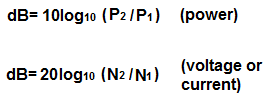﻿ Decibel (dB) Calculator

# Decibel (dB) CalculatorW

W

0.00dB

### Voltage or Current to Decibels (dB)

V or A

V or A

0.00dB

The Decibel (dB) calculator calculates the decibel gain (or loss) value from either a power ratio or a voltage/current ratio, according to the above formulas.

Decibels express the ratio between identical quantities. In electronics, the decibel is most often used to express the ratio between two powers, two voltages, or two currents.

A user enters in the two numbers to form the ratio and the result in unit decibels is automatically computed. Being that the decibels are calculated based on the ratio of two identical units (divided against each other), the calculation produces a unitless result. This means that a decibel calculation has no units. It is just a number.

Decibel calculation is useful for a slew of circuits. One example of a class of circuits which use this decibel calculation with extreme recurrence are audio circuits. When the level of two audio signals are taken and placed in a ratio over each other and the decibel value is calculated, we can then determine exactly how much louder or quieter one signal is compared to the other. If we place, for example, audio signal 1 is 30Vrms and audio signal 2 is 42.4Vrms, when we calculate out the decibel calculation, we get 3dB (decibels). A value of 3dB means that audio signal 2 is double the volume of audio signal 1, or taken the other way, audio signal 1 is one-half the power of audio signal 2. Decibel is a great way to reference signals. Once calculated and quantified, it has several points so that we can easily compare signals against each other. A difference of 3B means one signal is double the volume of the other, a difference of 6dB means 4 times the loudness, and so on. Every time we increase the db by 3, the volume difference doubles. This is why a difference of 6dB is 4 times as loud. And a difference of 9dB is 8 times as loud. This is why dB calculation is important, because it provides a easy reference when comparing signals.

Related Resources